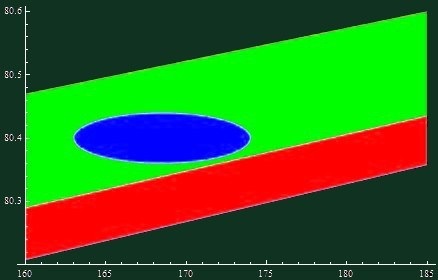Saturday, July 18, 2009

Top quarks and W bosons: MSSM is 13 times more likely than SM

Breaking news: LHC restart unfortunately moved to mid November
Tommaso Dorigo wrote another article in which he is trying to gradually turn his coat - from a supersymmetry hater to one of us, a champion of supersymmetry.

That's what everyone who cares about experiments is sometimes forced to do - except for the people who are always right, before the experiments are made. ;-) He talks about the ill-defined mass poles of the top quark and other technicalities. But the following chart - that we have already discussed in the past - is very comprehensible and very catchy:The plane is parameterized by "x", the top quark mass, and "y", the W boson mass, both in GeV.

The red region is the Standard Model (SM). The colors are taken from the traffic lights and red means "experimentally verifiable particle phenomenology stops in the 1970s". On the other hand, the green region is the Minimal Supersymmetric Standard Model (MSSM). The green color means "phenomenology will go on".

I neglected the thin region in the middle where they overlap.

The blue ellipse indicates the 68% confidence level region where the experimentally measured values of the top quark mass and the W boson mass probably lie. The carefully redefined (and lowered) top quark mass is centered around 168.5 GeV and the ellipse goes approximately 5.5 GeV in both directions. The W mass is centered around 80.4 GeV and the ellipse goes 0.04 GeV up and the same distance down: the W mass is much more accurately measured, of course. I neglected the slope of the ellipse if there was any: the ellipse looks like it's stretched exactly in the "x" direction.

Fine, how did I determine that the MSSM is 13 times more likely than the SM, using a simple Mathematica notebook? You see that the blue ellipse is fully located in the green, supersymmetric region. But what about the factor of 13?
Mathematica notebook, PDF preview
I parameterized the ellipses and those nearly straight lines for the SM and MSSM regions. Then I created a random generator following a normal distribution, rescaled in such a way that in approximately 68% of the cases, you remain inside the ellipse described above (that is approximated by a non-slanted one).

This random generator of points in the plane gave me about 96.5% of the points in the MSSM regions and 3.5% in the SM region. You may imagine that this generator simulates the possibilities (and their frequencies) that much more accurate experimenters will measure in the future, given the fuzzy knowledge we have today. Less than 0.1% were outside both strips, if you care. The green/red ratio between these two frequences is approximately 28: the MSSM wins.

But the prior probability density for the MSSM has to be lowered because the MSSM strip is about 2.2 times thicker, as measured around the 169 GeV top quark mass. In this sense, the MSSM is 2.2 times less predictive, the prior probability density has to be reduced relatively to the SM in order for the "total" prior probabilities of the SM and MSSM to be equal (a fair match!), and the ratio of probabilities for the MSSM and SM is therefore 28/2.2 which is close to 13.

Of course, this doesn't guarantee anything: 13 is not equal to infinity and unlike frequentist probabilities, Bayesian probabilities have no invariant scientific meaning because we're estimating the probability of an event that will only occur once. But it may give us a feeling how strongly the experiments are already trying to change our mind.